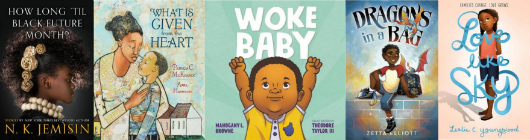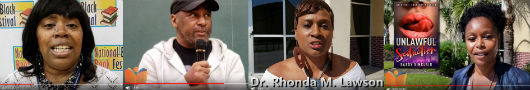Like   Tweet   Pin   in

## A Lucky Man: Stories by Jamel Brinkley

In a debut that announces the arrival of a significant new voice, A Lucky Man: Stories by Jamel Brinkley, reflects the tenderness and vulnerability of Black men and boys whose hopes sometimes betray them, especially in a world shaped by race, gender, and class―where luck may be the greatest fiction of all (Graywolf Press, May 08, 2018).

 table div table+table+table+table div table{width:100%;padding:0}table div table+table+table+table div table img{width:96.23%;padding:0;float:none}table div table+table+table+table div table td{width:100%;padding:0 1.88% 18px}/* styles */## The Poet X by Elizabeth Acevedo

Fans of Jacqueline Woodson, Meg Medina, and Jason Reynolds will fall hard for this novel-in-verse by an award-winning slam poet, about an Afro-Latina heroine who tells her story with blazing words and powerful truth (HarperTeen, Mar 06, 2018).

 table div table+table+table+table+table+table div table{width:100%;padding:0}table div table+table+table+table+table+table div table img{width:96.23%;padding:0;float:none}table div table+table+table+table+table+table div table td{width:100%;padding:0 1.88% 18px}/* styles *//* styles */ There too many wonderful new titles, by brilliant authors; Reader, I encourage you to visit our Recently Released Books page and our Upcoming Books page to the discover great new reads hand picked by AALBC just for you! We are constantly adding classic titles as well. You may research and discover great classic books by searching by category on our Books Homepage.
 table div table+table+table+table+table+table+table+table+table div table{width:100%;padding:0}table div table+table+table+table+table+table+table+table+table div table img{width:96.23%;padding:0;float:none}table div table+table+table+table+table+table+table+table+table div table td{width:100%;padding:0 1.88% 18px}/* styles */## Lighting the Fires of Freedom: African American Women in the Civil Rights Movement by Janet Dewart Bell

The book grew out of Dr. Bell’s dissertation on female civil rights activists. Through the interviews, Bell allows us to hear the voices of women who were unsung “sheroes” of the movement. They include women such as Leah Chase who provided a safe haven for civil rights activists in Dooky Chase, her New Orleans restaurant, Diane Nash, a leader of the Nashville Sit-in Movement, Dr. June Jackson Christmas, a trail-blazing psychologist who opened her Town House to civil rights workers, Aileen Hernandez who led student protests at Howard and later became a labor activist and leader of the National Organization for Women, Judy Richardson who was active in SNCC as a young leader and associate producer and education director for the documentary Eyes on the Prize, and Kathleen Cleaver, activist and professor of law (The New Press, May 8, 2018).

 table div table+table+table+table+table+table+table+table+table+table+table+table div table{width:100%;padding:0}table div table+table+table+table+table+table+table+table+table+table+table+table div table img{width:96.23%;padding:0;float:none}table div table+table+table+table+table+table+table+table+table+table+table+table div table td{width:100%;padding:0 1.88% 18px}/* styles */## The Talented Ribkins by Ladee Hubbard

Set in the south and using the literary elements of speculative fiction, Hubbard keeps readers riveted as they uncover the vulnerabilities, frailties and conflicts of the talented Ribkins. The concept of the maps brings to mind the tracks of the “underground railroad” in Colson Whitehead’s liberation narrative, The Underground Railroad. Just as the railroad provides a roadmap for those escaping enslavement, the maps provide a roadmap for those navigating the treacherous waters of a Jim Crow south (Melville House, Aug 08, 2017).

 table div table+table+table+table+table+table+table+table+table+table+table+table+table+table div table{width:100%;padding:0}table div table+table+table+table+table+table+table+table+table+table+table+table+table+table div table img{width:96.23%;padding:0;float:none}table div table+table+table+table+table+table+table+table+table+table+table+table+table+table div table td{width:100%;padding:0 1.88% 18px}/* styles */## Gay Girl, Good God: The Story of Who I Was, and Who God Has Always Been by Jackie Hill Perry

In the midst of arguably a time of the greatest push for the advocacy of LGBT acceptance, Jackie Hill-Perry has released a book entitled Gay Girl, Good God. This book is autobiographical in nature, and offers insight into her transformative journey of an unchanging God throughout her bondage to a life that was not pleasing to the God that she ambiguously knew prior to her transformation (B&H Publishing Group, Sep 3, 2018).

 table div table+table+table+table+table+table+table+table+table+table+table+table+table+table+table+table div table{width:100%;padding:0}table div table+table+table+table+table+table+table+table+table+table+table+table+table+table+table+table div table img{width:96.23%;padding:0;float:none}table div table+table+table+table+table+table+table+table+table+table+table+table+table+table+table+table div table td{width:100%;padding:0 1.88% 18px}/* styles */## Louise Thompson Patterson: A Life of Struggle for Justice by Keith Gilyard

Born in 1901, Louise Thompson Patterson was a dynamic and transformative radical Black woman who spent decades as a social activist.

“To study her story is to witness the courage, sacrifice, vision and discipline of someone who spent decades working to achieve justice and liberation for all people.” — Keith Gilyard (Duke University, Oct 5, 2017)

 table div table+table+table+table+table+table+table+table+table+table+table+table+table+table+table+table+table+table div table{width:100%;padding:0}table div table+table+table+table+table+table+table+table+table+table+table+table+table+table+table+table+table+table div table img{width:96.23%;padding:0;float:none}table div table+table+table+table+table+table+table+table+table+table+table+table+table+table+table+table+table+table div table td{width:100%;padding:0 1.88% 18px}/* styles */## Video Coverage of the National Black Book Festivaltable div table+table+table+table+table+table+table+table+table+table+table+table+table+table+table+table+table+table+table+table+table div table,table.module-20{width:41.51%;float:right;padding:0}table div table+table+table+table+table+table+table+table+table+table+table+table+table+table+table+table+table+table+table+table+table div table a{border:0 none;text-decoration:none}table div table+table+table+table+table+table+table+table+table+table+table+table+table+table+table+table+table+table+table+table+table div table img{width:100%!important;border:0 none;text-decoration:none}table div table+table+table+table+table+table+table+table+table+table+table+table+table+table+table+table+table+table+table+table+table div table td{width:100%;padding:0 0 20px 20px}/* styles */ The National Black Book Festival (NBBF) celebrated its 11th anniversary the last weekend in October. I attended the first NBBF in 2008, but have not been back since 2010 (check out some nice photos from that year). The event attracts a wide array of authors, publishers, book clubs, libraries, and book lovers from the all over the country. The festival was cofounded by author and entrepreneur Gwen Richardson and her husband Willie Richardson. I had the opportunity to video; longtime AALBC friend and bestselling author, Eric Jerome Dickey; erotic, romantic, suspense author, Sassy Sinclair (aka Pamela Samuels Young); author and founder of Horizons literary talk show, Dr. Rhonda M. Lawson; award winning, science fiction author Bill Liggins; debut author, Marq E. Redmond; and, of course, Gwen Richardson.
 table div table+table+table+table+table+table+table+table+table+table+table+table+table+table+table+table+table+table+table+table+table+table div table{width:100%;padding:0}table div table+table+table+table+table+table+table+table+table+table+table+table+table+table+table+table+table+table+table+table+table+table div table img{width:96.23%;padding:0;float:none}table div table+table+table+table+table+table+table+table+table+table+table+table+table+table+table+table+table+table+table+table+table+table div table td{width:100%;padding:0 1.88% 18px}/* styles */## Special Deals Directly from Book Publishers

 /* styles */ Each for the deals below are offered directly by two incredible, independent publishers, Black Classic Press and Blue Nile Press. They are just two of many institutions that keep Black literature vibrant, diverse, and true our voices. You won’t go wrong with either package. Consider sending them as gifts for the holidays.
 table div table+table+table+table+table+table+table+table+table+table+table+table+table+table+table+table+table+table+table+table+table+table+table+table+table div table,table.module-24{width:50.94%;float:right;padding:0}table div table+table+table+table+table+table+table+table+table+table+table+table+table+table+table+table+table+table+table+table+table+table+table+table+table div table a{border:0 none;text-decoration:none}table div table+table+table+table+table+table+table+table+table+table+table+table+table+table+table+table+table+table+table+table+table+table+table+table+table div table img{width:100%!important;border:0 none;text-decoration:none}table div table+table+table+table+table+table+table+table+table+table+table+table+table+table+table+table+table+table+table+table+table+table+table+table+table div table td{width:100%;padding:0 0 20px 20px}/* styles */ Blue Nile Press has given AALBC a great opportunity to sell these popular titles, together for the price of just one book! The Summer of my Fifteenth Year by Geri Spencer Hunter (read more) Raisins in Milk by David Covin (read more). Both books are excellent reads and AALBC Bestsellers. This deal is not available anywhere else!
 table div table+table+table+table+table+table+table+table+table+table+table+table+table+table+table+table+table+table+table+table+table+table+table+table+table+table div table{width:100%;padding:0}table div table+table+table+table+table+table+table+table+table+table+table+table+table+table+table+table+table+table+table+table+table+table+table+table+table+table div table img{width:96.23%;padding:0;float:none}table div table+table+table+table+table+table+table+table+table+table+table+table+table+table+table+table+table+table+table+table+table+table+table+table+table+table div table td{width:100%;padding:0 1.88% 18px}/* styles */table div table+table+table+table+table+table+table+table+table+table+table+table+table+table+table+table+table+table+table+table+table+table+table+table+table+table+table div table td,table.module-26{width:100%;padding:0}table div table+table+table+table+table+table+table+table+table+table+table+table+table+table+table+table+table+table+table+table+table+table+table+table+table+table+table div table{width:100%;float:none;margin-left:auto;margin-right:auto;padding:0}table div table+table+table+table+table+table+table+table+table+table+table+table+table+table+table+table+table+table+table+table+table+table+table+table+table+table+table div table a{border:0 none;text-decoration:none}table div table+table+table+table+table+table+table+table+table+table+table+table+table+table+table+table+table+table+table+table+table+table+table+table+table+table+table div table img{width:100%!important;border:0 none;text-decoration:none}/* styles */
 /* styles */ Black Classic Press is offering three eye-opening books which will make wonderful gifts or additions to your library. Speaking directly to African Americans after 9/11, What Next, by Walter Mosley, is a call to action for bringing about world peace. Readers will find that Mosley’s message rings true just as much today as it did 15 years ago when first published. In Fathering Words, acclaimed poet, E. Ethelbert Miller explores his development as an African American writer, the responsibility of his chosen career, and his ambitions to raise the consciousness of Black people. The Beautiful Struggle sheds light on the early days of the life of writer, thinker and 2015 National Book Award winner, Ta-Nehisi Coates in 1980s Baltimore. The discount will be applied at checkout and ends December 9, while supplies last.
 table div table+table+table+table+table+table+table+table+table+table+table+table+table+table+table+table+table+table+table+table+table+table+table+table+table+table+table+table+table div table{width:100%;padding:0}table div table+table+table+table+table+table+table+table+table+table+table+table+table+table+table+table+table+table+table+table+table+table+table+table+table+table+table+table+table div table img{width:96.23%;padding:0;float:none}table div table+table+table+table+table+table+table+table+table+table+table+table+table+table+table+table+table+table+table+table+table+table+table+table+table+table+table+table+table div table td{width:100%;padding:0 1.88% 18px}/* styles */## AALBC Online Book Club — December’s Selection

 table div table+table+table+table+table+table+table+table+table+table+table+table+table+table+table+table+table+table+table+table+table+table+table+table+table+table+table+table+table+table+table div table,table.module-30{width:33.96%;float:right;padding:0}table div table+table+table+table+table+table+table+table+table+table+table+table+table+table+table+table+table+table+table+table+table+table+table+table+table+table+table+table+table+table+table div table a{border:0 none;text-decoration:none}table div table+table+table+table+table+table+table+table+table+table+table+table+table+table+table+table+table+table+table+table+table+table+table+table+table+table+table+table+table+table+table div table img{width:100%!important;border:0 none;text-decoration:none}table div table+table+table+table+table+table+table+table+table+table+table+table+table+table+table+table+table+table+table+table+table+table+table+table+table+table+table+table+table+table+table div table td{width:100%;padding:0 0 20px 20px}/* styles */ Participate in our Book Club’s Discussion, of Stamped from the Beginning: The Definitive History of Racist Ideas in America by Ibram X. Kendi, during the entire month of December 2018 In Stamped from the Beginning, the 2016 National Book Award winner for Nonfiction, historian Ibram X. Kendi argues some Americans cling desperately to the myth that we are living in a post-racial society, that the election of the first Black president spelled the doom of racism. In fact, racist thought is alive and well in America — more sophisticated and more insidious than ever and we must first understand how ﻿racist ideas were developed, disseminated, and enshrined in American society. To participate you’ll need to sign up. Please visit this page: https://aalbc.com/tc/ click the “Sign Up” link on the top right hand side of the page; you’ll just need to enter valid email address (which will never be shared) and the name you would like to use. I’ll approve your account, and you can participate in the conversation taking place here this month. Tony Lindsay and Doriel Larrier are the book club’s moderators. Also, check out our reading list for the remainder of the year.
 table div table+table+table+table+table+table+table+table+table+table+table+table+table+table+table+table+table+table+table+table+table+table+table+table+table+table+table+table+table+table+table+table div table{width:100%;padding:0}table div table+table+table+table+table+table+table+table+table+table+table+table+table+table+table+table+table+table+table+table+table+table+table+table+table+table+table+table+table+table+table+table div table img{width:96.23%;padding:0;float:none}table div table+table+table+table+table+table+table+table+table+table+table+table+table+table+table+table+table+table+table+table+table+table+table+table+table+table+table+table+table+table+table+table div table td{width:100%;padding:0 1.88% 18px}/* styles */table div table+table+table+table+table+table+table+table+table+table+table+table+table+table+table+table+table+table+table+table+table+table+table+table+table+table+table+table+table+table+table+table+table div table,table.module-32{width:26.42%;float:left;padding:0}table div table+table+table+table+table+table+table+table+table+table+table+table+table+table+table+table+table+table+table+table+table+table+table+table+table+table+table+table+table+table+table+table+table div table a{border:0 none;text-decoration:none}table div table+table+table+table+table+table+table+table+table+table+table+table+table+table+table+table+table+table+table+table+table+table+table+table+table+table+table+table+table+table+table+table+table div table img{width:100%!important;border:0 none;text-decoration:none}table div table+table+table+table+table+table+table+table+table+table+table+table+table+table+table+table+table+table+table+table+table+table+table+table+table+table+table+table+table+table+table+table+table div table td{width:100%;padding:0 20px 20px 0}/* styles */ Dear Reader, As always AALBC continues to thrive because of your patronage and support. If you value what we do, please purchase your subscription to AALBC's eNewsletter. If you have already done so, accept my personal thank you, and a thank you for the authors whose work you help support. Finally, when you discover something of value on AALBC please be sure to share the information with others.
 /* styles */ Peace and Love, Troy Johnson Founder & Webmaster, AALBC.com
 table div table+table+table+table+table+table+table+table+table+table+table+table+table+table+table+table+table+table+table+table+table+table+table+table+table+table+table+table+table+table+table+table+table+table+table div table,table.module-34{width:27.55%;float:left;padding:0}table div table+table+table+table+table+table+table+table+table+table+table+table+table+table+table+table+table+table+table+table+table+table+table+table+table+table+table+table+table+table+table+table+table+table+table div table a{border:0 none;text-decoration:none}table div table+table+table+table+table+table+table+table+table+table+table+table+table+table+table+table+table+table+table+table+table+table+table+table+table+table+table+table+table+table+table+table+table+table+table div table img{width:100%!important;border:0 none;text-decoration:none}table div table+table+table+table+table+table+table+table+table+table+table+table+table+table+table+table+table+table+table+table+table+table+table+table+table+table+table+table+table+table+table+table+table+table+table div table td{width:100%;padding:0 20px 20px 0}/* styles */
 /* styles */ Our newsletter may be read on your Kindle ebook reader or via RSS. Consider sponsoring our eNewsletter or a dedicated mailing.
 table div table+table+table+table+table+table+table+table+table+table+table+table+table+table+table+table+table+table+table+table+table+table+table+table+table+table+table+table+table+table+table+table+table+table+table+table+table div table td,table.module-36{width:100%;padding:0}table div table+table+table+table+table+table+table+table+table+table+table+table+table+table+table+table+table+table+table+table+table+table+table+table+table+table+table+table+table+table+table+table+table+table+table+table+table div table{width:100%;float:none;margin-left:auto;margin-right:auto;padding:0}table div table+table+table+table+table+table+table+table+table+table+table+table+table+table+table+table+table+table+table+table+table+table+table+table+table+table+table+table+table+table+table+table+table+table+table+table+table div table a{border:0 none;text-decoration:none}table div table+table+table+table+table+table+table+table+table+table+table+table+table+table+table+table+table+table+table+table+table+table+table+table+table+table+table+table+table+table+table+table+table+table+table+table+table div table img{width:100%!important;border:0 none;text-decoration:none}/* styles */
 /* styles */ ★ AALBC.com eNewsletter – November 27, 2018 - Issue #264